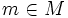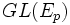# Difference between revisions of "Holonomy group"

## Definition

Let$M$ be a differential manifold,$E$ a vector bundle over$M$ and$\nabla$ a connection for$E$. For a point$m \in M$ the holonomy group at$m$ is the subgroup of$GL(E_p)$ comprising those linear transformations that arise as the holonomy of a loop at$m$.

If the differential manifold is path-connected, the holonomy groups at distinct points are conjugate as subgroups of$GL(E_p)$ so we can talk of the holonomy group.

## Facts

### Reduction of structure group to holonomy group

Any path-connected differential manifold can be treated as a principal bundle with structure group being the holonomy group. The description is as follows:

• Pick (arbitrarily) a basis at a particular point$p$
• Now, for each point$m$, the fibre at that point is the set of all bases at$m$ that can arise from the basis at$p$ by means of transport along a curve using the connection$\nabla$.
• In particular, any two bases at$m$ differ by the holonomy of a loop, which lies in$H$. Thus$H$ acts freely and transitively on the fibre at each point.

Moreover the holonomy group is the smallest group to which the structure group can be reduced (for the same reasons).